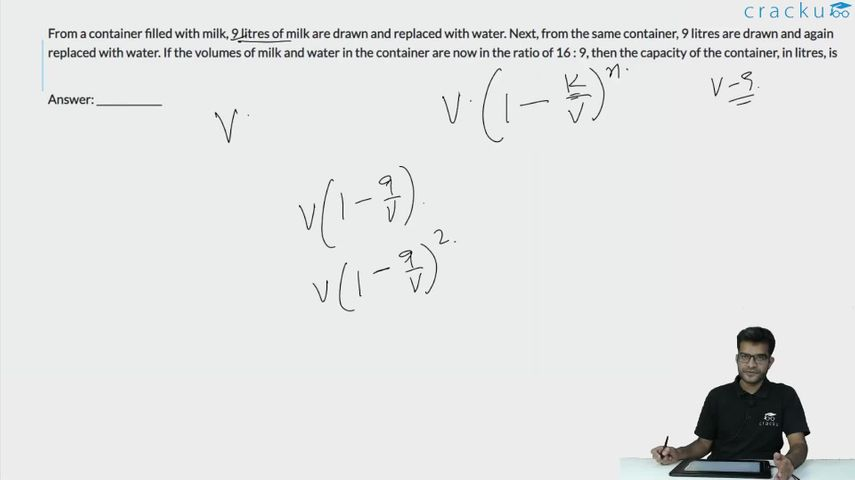Question 52

# From a container filled with milk, 9 litres of milk are drawn and replaced with water. Next, from the same container, 9 litres are drawn and again replaced with water. If the volumes of milk and water in the container are now in the ratio of 16 : 9, then the capacity of the container, in litres, is

Solution

Let initial volume be V, final be F for milk.

The formula is given by : $$F\ =\ V\cdot\left(1-\frac{K}{V}\right)^n$$ n is the number of times the milk is drawn and replaced.

so we get $$F=\ V\left(1-\frac{K}{V}\right)^{^2}$$
here K =9
we get
$$\frac{16}{25}V\ =\ V\ \left(1-\frac{9}{V}\right)^{^2}$$
we get $$1-\frac{9}{V}=\ \frac{4}{5}or\ -\frac{4}{5}$$

If considering $$1-\frac{9}{V}=-\frac{4}{5}$$

V =5, but this is not possible because 9 liters is drawn every time.

Hence : $$1-\frac{9}{V}=\frac{4}{5},\ V\ =\ 45\ liters$$

### View Video Solution